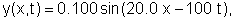# 1-5 Speed of a sinusoidal traveling waveA transverse harmonic wave traveling on a string is described bywhere x and y are in meters and t is in seconds.

1. What is the speed of the wave?
2. Find an expression for the transverse velocity of an element of the string located at position x and at time t.
3. What is the maximum transverse speed? Where does it occur?
4. Find an expression for the transverse acceleration of an element of the string located at position x and at time t.
5. What is the maximum transverse acceleration? Where does it occur?
Example 3